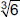# User Forum

Subject :IMO    Class : Class 8

## Ans 1:

Class : Class 8
0 is both a number and the numerical digit used to represent that number in numerals. The number 0 fulfills a central role in mathematics as the additive identity of the integers, real numbers, and many other algebraic structures. As a digit, 0 is used as a placeholder in place value systems.

Subject :IMO    Class : Class 8

## Ans 1:

Class : Class 8
0 is both a number and the numerical digit used to represent that number in numerals. The number 0 fulfills a central role in mathematics as the additive identity of the integers, real numbers, and many other algebraic structures. As a digit, 0 is used as a placeholder in place value systems.

Subject :IMO    Class : Class 8

Class : Class 1

We have,Subject :IMO    Class : Class 3

Class : Class 1

1000 in roman numerals = M

Subject :IMO    Class : Class 4

Class : Class 1

Number of detective books he read
= 4 × 5 = 20.

Class : Class 5
Ans. C (20)

## Ans 3:

Class : Class 5
20

Subject :IMO    Class : Class 5

Class : Class 1

The correct answer is B - 17766

Rounding off rounds off 17766 to the nearest thousand, you will get 18000.

Rounding off 17766  to the nearest hundred, you will get 17800.

And rounding off to the nearest ten, you will get 17770.

Class : Class 6
B

## Ans 3:

Class : Class 6
(B) 17766 When 17766 is rounded to the nearest tens, it is 17770 When 17766 is rounded to the nearest hundreds, it is 178000 When 17766 is rounded to the nearest thousands, it is 18000 Therefore, 17766 agrees with all the rules and is the right answer.

Class : Class 4

Class : Class 5
B

## Ans 6:

Class : Class 6
(B) 17766 When 17766 is rounded to the nearest tens, it is 17770 When 17766 is rounded to the nearest hundreds, it is 178000 When 17766 is rounded to the nearest thousands, it is 18000 Therefore, 17766 agrees with all the rules and is the right answer.

Subject :IMO    Class : Class 9

Class : Class 1## Ans 2:

Class : Class 10
D

Subject :IMO    Class : Class 4

Class : Class 1

Subject :IMO    Class : Class 5

## Ans 1:

Class : Class 5
I still donât understand how the answer is C. I got the answer as 14

Class : Class 5

## Ans 3:

Class : Class 3
teree to pit jaega

Class : Class 5

Class : Class 6

Class : Class 5

## Ans 7:

Class : Class 5
But I agree that the answer is B

## Ans 8:

Class : Class 5

Subject :IMO    Class : Class 6

## Ans 1:

Class : Class 6
x =20+6-5-25= - 4. so, x = - 4

Class : Class 6

## Ans 3:

Class : Class 4
Like this :- First we will remove 6 from the left side of the equation and add 6 on the right side this would bring this :- x +25+5=26 Then we will substract 25 from both sides ,this would bring this :- x + 5 = 1 Then we will substract 5 from both sides this will give us :- x = -4 ,so x = -4

Class : Class 7
-4